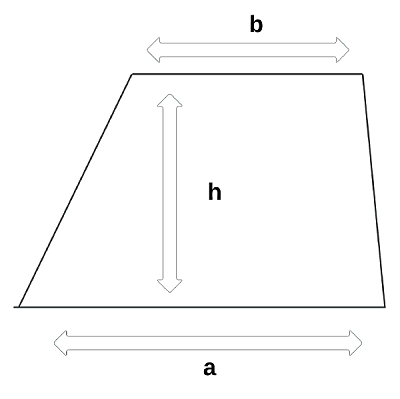# Haskell Program to Find the Area of a Trapezium

This tutorial discusses writing a program to print the area of a trapezium in the Haskell programming language.

A trapezium is a quadrilateral with one opposite side parallel.The above figure is a trapezium. The length of one of the parallel sides is denoted by a and the other side is denoted by b. The height of the trapezium is denoted by h.

The area of a trapezium is defined as (a+b)/(2*h), where a and b are the lengths of the parallel sides and h is the height or distance between the parallel sides.

In this tutorial, we see two ways to implement a program to find the area of the trapezium.

• Program to find the area of the trapezium in the main function.
• Program to find the area of the trapezium using a custom function.

## Algorithm Steps

• Take input or initialize the variables.
• Implement the program logic to compute the area of the trapezium.
• Print or display the area.

## Example 1

Program to find the area of the trapezium in the main function

main :: IO()
main = do
-- declaring and initializing variables for sides and height
let a = 5
let b = 6
let h = 8
-- computing the area
let area = 0.5*(a+b)*h
-- printing or displaying the area
print ("The area of the trapezium with parallel sides "++ show a ++ "," ++ show b ++ " and height " ++ show h ++ " is:")
print (area)


## Output

"The area of the trapezium with parallel sides 5.0,6.0 and height 8.0 is:"
44.0


In the above program, we declared and initialized variables for sides and height as a, b, and h respectively with values 5, 6, and 8. We computed the area of the trapezium using the appropriate formula using the variables and loaded it into a variable area. Finally, printed the area using the print function.

Note − The function show takes the argument a number and returns the parsed string of a number. “++” is an operator to concatenate strings in Haskell.

## Example 2

Program to find the area of the trapezium using a custom function

-- function declaration
area :: Float->Float->Float->Float
-- function definition
area a b h =  0.5*(a+b)*h
main :: IO()
main = do
-- declaring and initializing variables for sides and height
let a = 5
let b = 6
let h = 8
-- computing the area
let d = area a b h
-- printing or displaying the area
print ("The area of the trapezium with parallel sides "++ show a ++ "," ++ show b ++ " and height " ++ show h ++ " is:")
print (d)


## Output

"The area of the trapezium with parallel sides 5.0,6.0 and height 8.0 is:"
44.0


In the above program, we declared a function area as such it takes three float arguments and returns a float. In its function definition, three arguments are accepted a, b, and c. In this area of the trapezium is computed and returned. In the main function, we declared and initialized variables for sides and height as a, b, and h respectively with values 5, 6, and 8. We invoked the function area with a, b, and c as arguments and loaded the returned output into a variable d. Finally, printed the area using the print function.

## Conclusion

In this tutorial, we discussed different ways to implement the program to compute the area of the trapezium in the Haskell programming language.